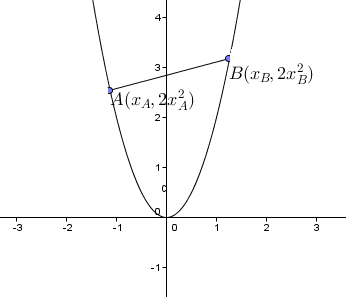# 2-dimensional bowlThe ends $A$ and $B$ of a rod of length of $\sqrt{5}$ are sliding along the curve $y=2x^2$. Let $x_A$ and $x_B$ be the $x$-coordinates of the ends. At the moment when $A$ is at $(0,0)$ and $B$ is at $(1,2)$. Find the value of the derivative $\dfrac{dx_B}{dx_A}$.

×